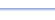Program: PROPORTN

PROPORTN

• Finds the missing value in a direct or inverse proportion, given the other three values.

Useful for:

• SAT
• ACT
• Algebra 1
• Algebra 2
• Geometry

See full list of programs

Try these problems using PROPORTN:
on MathPro Q&A Forum
in SAT and ACT prep books

Example 1

Q: x varies directly with y.  If x=4 when y=5, what is y when x is 7?

Solution:

Run PROPORTN.  How?

Enter the first pair
of x and y values
as shown:

Select choice 1, that
you have the second
x-value.

Enter x2 = 7.

Choose 1 because
the question says
x and y vary directly.

The program reports:

Interpretation:
y = 8.75

See Example 2